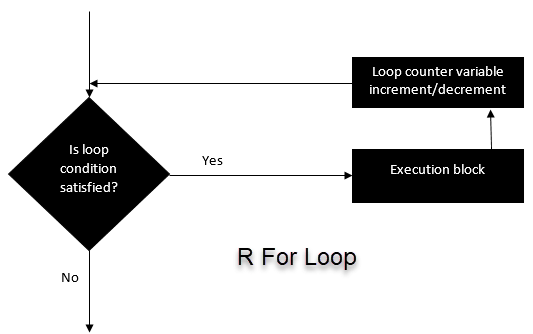# For Loop in R with Examples for List and Matrix

A for loop is very valuable when we need to iterate over a list of elements or a range of numbers. Loop can be used to iterate over a list, data frame, vector, matrix or any other object. The braces and square bracket are compulsory.

## For Loop Syntax and Examples

For (i in vector) { Exp }

Here,

R will loop over all the variables in vector and do the computation written inside the exp.Let’s see a few examples.

Example 1 : We iterate over all the elements of a vector and print the current value.

Learn Java Programming with Beginners Tutorial

# Create fruit vector fruit <- c(‘Apple’, ‘Orange’, ‘Passion fruit’, ‘Banana’) # Create the for statement for ( i in fruit){ print(i) }

Output:

##  “Apple” ##  “Orange” ##  “Passion fruit” ##  “Banana”

Example 2 : creates a non-linear function by using the polynomial of x between 1 and 4 and we store it in a list

# Create an empty list list <- c() # Create a for statement to populate the list for (i in seq(1, 4, by=1)) { list[[i]] <- i*i } print(list)

Output:

##  1 4 9 16

The for loop is very valuable for machine learning tasks. After we have trained a model, we need to regularize the model to avoid over-fitting. Regularization is a very tedious task because we need to find the value that minimizes the loss function. To help us detect those values, we can make use of a for loop to iterate over a range of values and define the best candidate.

## For Loop over a list

Looping over a list is just as easy and convenient as looping over a vector. Let’s see an example

# Create a list with three vectors fruit <- list(Basket = c(‘Apple’, ‘Orange’, ‘Passion fruit’, ‘Banana’), Money = c(10, 12, 15), purchase = FALSE) for (p in fruit) { print§ }

Output:

## For Loop over a matrix

A matrix has 2-dimension, rows and columns. To iterate over a matrix, we have to define two for loop, namely one for the rows and another for the column.

Output: